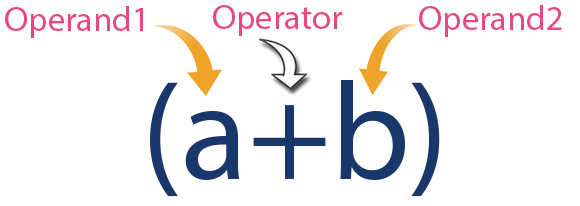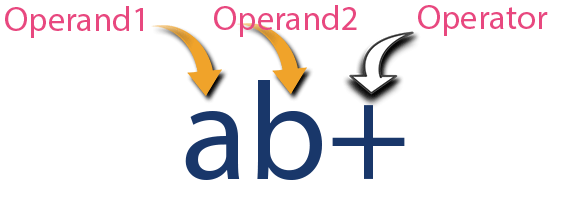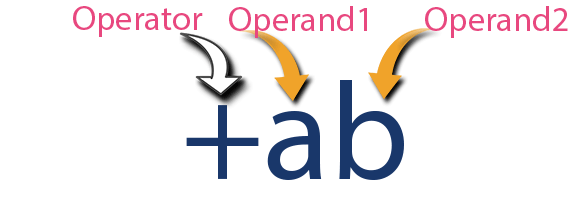The perfect place for easy learning...

×

# What is an expression?

In any programming language, if we want to perform any calculation or to frame any condition etc., we use a set of symbols to perform the task. These set of symbols makes an expression.
In the C programming language, an expression is defined as follows.

An expression is a collection of operators and operands that represents a specific value.

In the above definition, an operator is a symbol that performs tasks like arithmetic operations, logical operations, and conditional operations, etc.
Operands are the values on which the operators perform the task. Here operand can be a direct value or variable or address of memory location.

# Expression Types in C

In the C programming language, expressions are divided into THREE types. They are as follows...

1. Infix Expression
2. Postfix Expression
3. Prefix Expression

The above classification is based on the operator position in the expression.

# Infix Expression

The expression in which the operator is used between operands is called infix expression.
The infix expression has the following general structure.

Operand1 Operator Operand2

Example# Postfix Expression

The expression in which the operator is used after operands is called postfix expression.
The postfix expression has the following general structure.

Operand1 Operand2 Operator

Example# Prefix Expression

The expression in which the operator is used before operands is called a prefix expression.
The prefix expression has the following general structure.

Operator Operand1 Operand2

Example